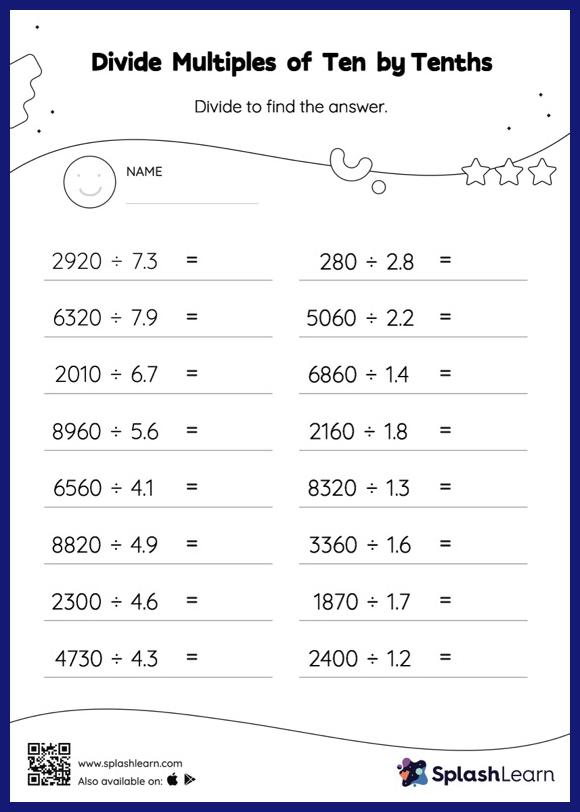# Divide Multiples of Ten by Tenths without Remainder: Horizontal Division Worksheet

Home > Divide Multiples of Ten by Tenths without Remainder: Horizontal DivisionThis worksheet challenges young mathematicians to hone their math skills by solving a set of problems on dividing multiples of ten by tenths without remainder. When dividing a number by a decimal, students divide without considering the decimal point and then move each digit of the quotient to the left based on the number of decimal digits in the divisor. Divide multiples of ten by tenths without remainder provides plenty of practice with this concept. In this worksheet, students practice solving problems written in the horizontal format. How numbers are laid out in a problem affects the method a student employs to solve it. Therefore to develop actual fluency and mastery of multiple strategies, students must practice different formats.